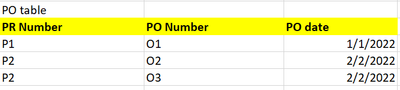cancel
Showing results for
Did you mean:Anonymous
Not applicable

## how to find the datediff between 2 table that has a indirect relationship

Hi everyone, hope you doing well.

I have face some difficulties in finding the number of date difference between 2 table that has a indirect relationship.

For example, PR table have a direct relationship to PO table, and PO table have a direct relationship to GRN table.

how can I find out the datediff between PR date ( in PR table) and GRN Date (in GRN table).thank you

1 ACCEPTED SOLUTIONCommunity Support

Hi @Anonymous ,

I suggest you to create a measure to calculate the datediff between PR date and GRN date. Here I create a sample to have a test.

Data model:Measure:

``````Datediff =
VAR _SUMMARIZE =
SUMMARIZE (
GRN,
GRN[GRN Number],
GRN[GRN date],
"Datediff",
VAR _PR_NUMBER =
CALCULATE ( MAX ( PO[PR Number] ) )
VAR _PR_DATE =
CALCULATE ( MAX ( PR[PR date] ), FILTER ( PR, PR[PR Number] = _PR_NUMBER ) )
RETURN
DATEDIFF ( _PR_DATE, [GRN date], DAY )
)
RETURN
SUMX(_SUMMARIZE,[Datediff])``````

Result is as below.Best Regards,
Rico Zhou

If this post helps, then please consider Accept it as the solution to help the other members find it more quickly.

5 REPLIES 5Community Support

Hi @Anonymous ,

I suggest you to create a measure to calculate the datediff between PR date and GRN date. Here I create a sample to have a test.

Data model:Measure:

``````Datediff =
VAR _SUMMARIZE =
SUMMARIZE (
GRN,
GRN[GRN Number],
GRN[GRN date],
"Datediff",
VAR _PR_NUMBER =
CALCULATE ( MAX ( PO[PR Number] ) )
VAR _PR_DATE =
CALCULATE ( MAX ( PR[PR date] ), FILTER ( PR, PR[PR Number] = _PR_NUMBER ) )
RETURN
DATEDIFF ( _PR_DATE, [GRN date], DAY )
)
RETURN
SUMX(_SUMMARIZE,[Datediff])``````

Result is as below.Best Regards,
Rico Zhou

If this post helps, then please consider Accept it as the solution to help the other members find it more quickly.Anonymous
Not applicable

okay, got it! thank you so much for the clarification. really appreciate thatSuper User

If the relationships are both one-to-one then you can use the RELATED function. e.g. you could add a column to the PR table like

``Date diff = DATEDIFF('PR table'[PR date], RELATED( 'GRN table'[GRN Date]), DAY)``Anonymous
Not applicable

Hi @johnt75 ,
But both of the relationship is one-to-many in this case. is thre an alternative way to solve this ?Super User

You could try

``````Date diff =
var prNumber = 'PR Table'[PR Number]
var poNumber = SELECTCOLUMNS( TOPN(1,
FILTER( 'PO Table', 'PO Table'[PR Number] = prNumber),
'PO Table'[PO Date]),
"@val", 'PO Table'[PO Number])
var grnDate = SELECTCOLUMNS( TOPN(1,
FILTER( 'GRN Table', 'GRN Table'[PO Number] = poNumber),
'GRN Table'[GRN Date]),
"@val2", 'GRN Table'[GRN Date])
return DATEDIFF( 'PR Table'[PR Date], grnDate, DAY)``````

This relies on there being only 1 entry per PO or per GRN per date. If there can be multiple entries for a given date then you will need to use another column for the sorting in the TOPN statements which is guaranteed to be unique. If necessary, you can use Power Query to generate an index column during data import